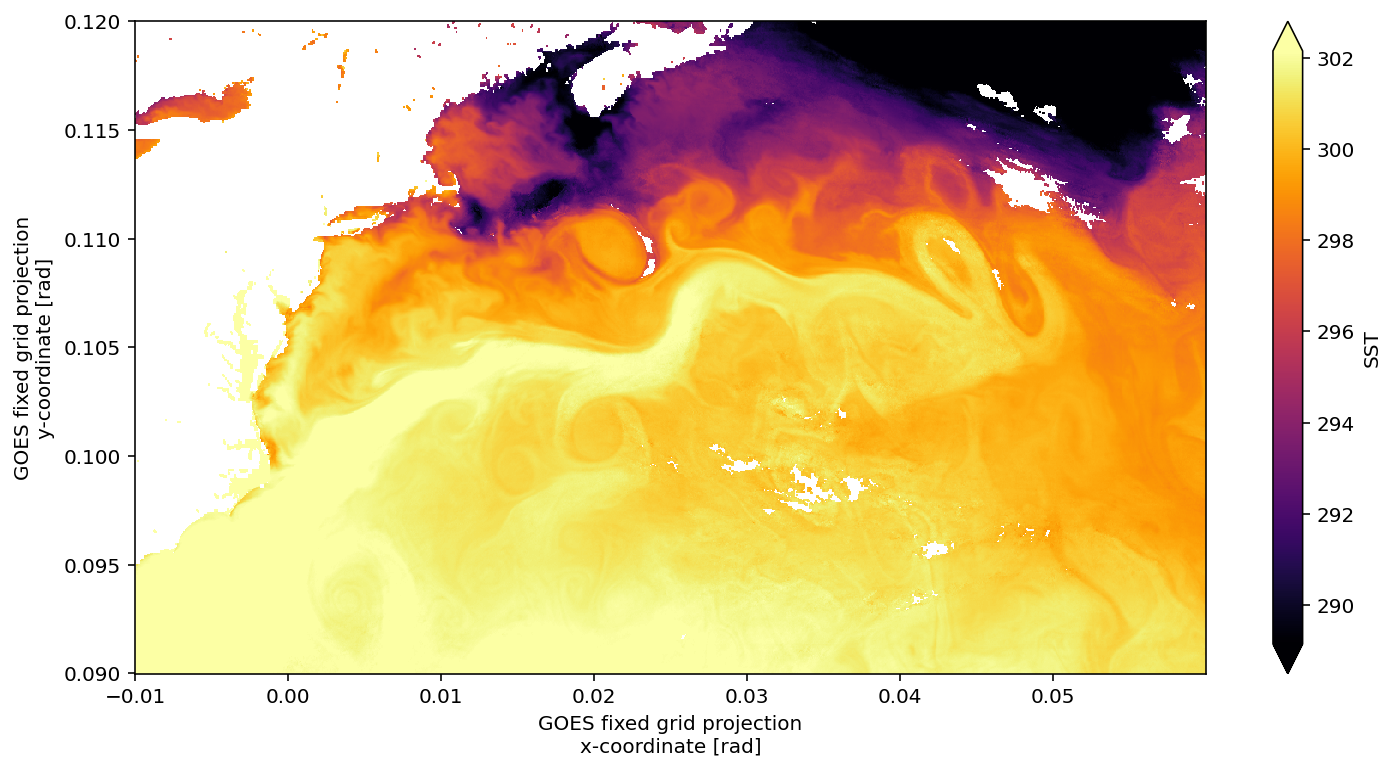# Comparison reading GOES-R data from AWS S3 in netCDF versus zarr¶

• Funding: Interagency Implementation and Advanced Concepts Team IMPACT for the Earth Science Data Systems (ESDS) program and AWS Public Dataset Program
• Software developed for OceanHackWeek 2020

## Why data format matters¶

• NetCDF sprinkles metadata throughout files, making them slow to access and read data
• Zarr consolidates the metadata, making them FAST for access and reading # Comparison reading GOES data from AWS S3 in netCDF versus zarr
In :
#libraries
import datetime as dt
import xarray as xr
import fsspec
import s3fs
import os.path
import matplotlib.pyplot as plt

# make datasets display nicely
xr.set_options(display_style="html")

#magic fncts #put static images of your plot embedded in the notebook
%matplotlib inline
plt.rcParams['figure.figsize'] = 12, 6
%config InlineBackend.figure_format = 'retina'


## Define a function to read the netCDF data¶

• The last 30 days are on S3 standard access, after that it is move to infrequent access
• It takes about 3 minutes to connect to a days worth of data for a single product
In :
def get_geo_data(sat,lyr,idyjl):
# arguments
# sat   goes-east,goes-west,himawari
# lyr   year
# idyjl day of year

d = dt.datetime(lyr,1,1) + dt.timedelta(days=idyjl)
fs = s3fs.S3FileSystem(anon=True) #connect to s3 bucket!

#create strings for the year and julian day
imon,idym=d.month,d.day
syr,sjdy,smon,sdym = str(lyr).zfill(4),str(idyjl).zfill(3),str(imon).zfill(2),str(idym).zfill(2)

#use glob to list all the files in the directory
if sat=='goes-east':
file_location,var = fs.glob('s3://noaa-goes16/ABI-L2-SSTF/'+syr+'/'+sjdy+'/*/*.nc'),'SST'
if sat=='goes-west':
file_location,var = fs.glob('s3://noaa-goes17/ABI-L2-SSTF/'+syr+'/'+sjdy+'/*/*.nc'),'SST'
if sat=='himawari':
file_location,var = fs.glob('s3://noaa-himawari8/AHI-L2-FLDK-SST/'+syr+'/'+smon+'/'+sdym+'/*/*L2P*.nc'),'sea_surface_temperature'

#make a list of links to the file keys
if len(file_location)<1:
return file_ob
file_ob = [fs.open(file) for file in file_location]        #open connection to files

#open all the day's data
ds = xr.open_mfdataset(file_ob,combine='nested',concat_dim='time') #note file is super messed up formatting

#clean up coordinates which are a MESS in GOES
#rename one of the coordinates that doesn't match a dim & should
if not sat=='himawari':
ds = ds.rename({'t':'time'})
ds = ds.reset_coords()
else:
ds = ds.rename({'ni':'x','nj':'y'}) #for himawari change dims to match goes

#put in to Celsius
#ds[var] -= 273.15   #nice python shortcut to +- from itself a-=273.15 is the same as a=a-273.15
#ds[var].attrs['units'] = '$^\circ$C'

return ds


## Open a day of GOES-16 (East Coast) Data and plot the average SST - netCDF¶

In :
%%time

lyr, idyjl = 2020, 210

ds = get_geo_data('goes-east',lyr,idyjl)

CPU times: user 15.2 s, sys: 2.31 s, total: 17.5 s
Wall time: 2min 45s

In :
%%time
subset = ds.sel(x=slice(-0.01,0.06),y=slice(0.12,0.09))  #reduce to GS region
mean_dy = masked.mean('time',skipna=True)   #here I want all possible values so skipna=True
mean_dy.plot(vmin=16+273.15,vmax=29+273.15,cmap='inferno')

CPU times: user 1min 46s, sys: 34.1 s, total: 2min 20s
Wall time: 19min 19s

/srv/conda/envs/notebook/lib/python3.8/site-packages/dask/array/numpy_compat.py:40: RuntimeWarning: invalid value encountered in true_divide
x = np.divide(x1, x2, out)

Out:
<matplotlib.collections.QuadMesh at 0x7f5e4d9bbf10># Exact same process, but with Zarr file stored on S3 us-east-1, just like the netcdf files above¶

In :
%%time

file_location = 's3://ohw-bucket-us-east-1/goes_zarr'

ikey = fsspec.get_mapper(file_location,anon=False)

ds = xr.open_zarr(ikey)

ds

CPU times: user 967 ms, sys: 0 ns, total: 967 ms
Wall time: 24.1 s

Out:
<xarray.Dataset>
Dimensions:                                                 (SST_day_night_emissive_bands: 4, SST_night_only_emissive_band: 1, number_of_LZA_bounds: 2, number_of_SZA_bounds: 2, number_of_image_bounds: 2, number_of_time_bounds: 2, time: 24, x: 5424, y: 5424)
Coordinates:
* time                                                    (time) datetime64[ns] ...
* x                                                       (x) float32 -0.15...
* y                                                       (y) float32 0.151...
Dimensions without coordinates: SST_day_night_emissive_bands, SST_night_only_emissive_band, number_of_LZA_bounds, number_of_SZA_bounds, number_of_image_bounds, number_of_time_bounds
Data variables:
DQF                                                     (time, y, x) float32 dask.array<chunksize=(1, 5424, 5424), meta=np.ndarray>
SST                                                     (time, y, x) float32 dask.array<chunksize=(1, 5424, 5424), meta=np.ndarray>
day_solar_zenith_angle                                  float32 ...
day_solar_zenith_angle_bounds                           (time, number_of_SZA_bounds) float32 dask.array<chunksize=(1, 2), meta=np.ndarray>
night_solar_zenith_angle                                float32 ...
night_solar_zenith_angle_bounds                         (time, number_of_SZA_bounds) float32 dask.array<chunksize=(1, 2), meta=np.ndarray>
quantitative_local_zenith_angle                         float32 ...
quantitative_local_zenith_angle_bounds                  (time, number_of_LZA_bounds) float32 dask.array<chunksize=(1, 2), meta=np.ndarray>
retrieval_local_zenith_angle                            float32 ...
retrieval_local_zenith_angle_bounds                     (time, number_of_LZA_bounds) float32 dask.array<chunksize=(1, 2), meta=np.ndarray>
retrieval_solar_zenith_angle                            float32 ...
retrieval_solar_zenith_angle_bounds                     (time, number_of_SZA_bounds) float32 dask.array<chunksize=(1, 2), meta=np.ndarray>
time_bounds                                             (time, number_of_time_bounds) datetime64[ns] dask.array<chunksize=(24, 2), meta=np.ndarray>
twilight_solar_zenith_angle_bounds                      (time, number_of_SZA_bounds) float32 dask.array<chunksize=(1, 2), meta=np.ndarray>
x_image                                                 float32 ...
x_image_bounds                                          (time, number_of_image_bounds) float32 dask.array<chunksize=(1, 2), meta=np.ndarray>
y_image                                                 float32 ...
y_image_bounds                                          (time, number_of_image_bounds) float32 dask.array<chunksize=(1, 2), meta=np.ndarray>
Attributes:
Conventions:               CF-1.7
_FillValue:                [-999.0]
cdm_data_type:             Image
cell_methods:              quantitative_local_zenith_angle: sum retrieval...
dataset_name:              OR_ABI-L2-SSTF-M6_G16_s20202100000205_e2020210...
date_created:              2020-07-28T01:05:45.6Z
grid_mapping:              goes_imager_projection
id:                        14a121c9-41b8-4552-9c28-69e6bcd4952f
institution:               DOC/NOAA/NESDIS > U.S. Department of Commerce,...
instrument_ID:             FM1
instrument_type:           GOES R Series Advanced Baseline Imager
keywords:                  OCEANS > OCEAN TEMPERATURE > SEA SURFACE TEMPE...
keywords_vocabulary:       NASA Global Change Master Directory (GCMD) Ear...
license:                   Unclassified data.  Access is restricted to ap...
long_name:                 standard deviation of the difference of the ob...
naming_authority:          gov.nesdis.noaa
orbital_slot:              GOES-East
platform_ID:               G16
processing_level:          National Aeronautics and Space Administration ...
production_data_source:    Realtime
production_environment:    OE
production_site:           NSOF
project:                   GOES
scene_id:                  Full Disk
standard_name_vocabulary:  CF Standard Name Table (v35, 20 July 2016)
summary:                   The ABI Sea Surface Temperature (SST) is calcu...
time_coverage_end:         2020-07-28T00:59:51.3Z
time_coverage_start:       2020-07-28T00:00:20.5Z
timeline_id:               ABI Mode 6
title:                     ABI L2 Sea Surface (Skin) Temperature
units:                     K
In :
%%time
subset = ds.sel(x=slice(-0.01,0.06),y=slice(0.12,0.09))  #reduce to GS region

CPU times: user 3.03 s, sys: 1.8 s, total: 4.83 s

/srv/conda/envs/notebook/lib/python3.8/site-packages/dask/array/numpy_compat.py:40: RuntimeWarning: invalid value encountered in true_divide

<matplotlib.collections.QuadMesh at 0x7f5e4ce46eb0>